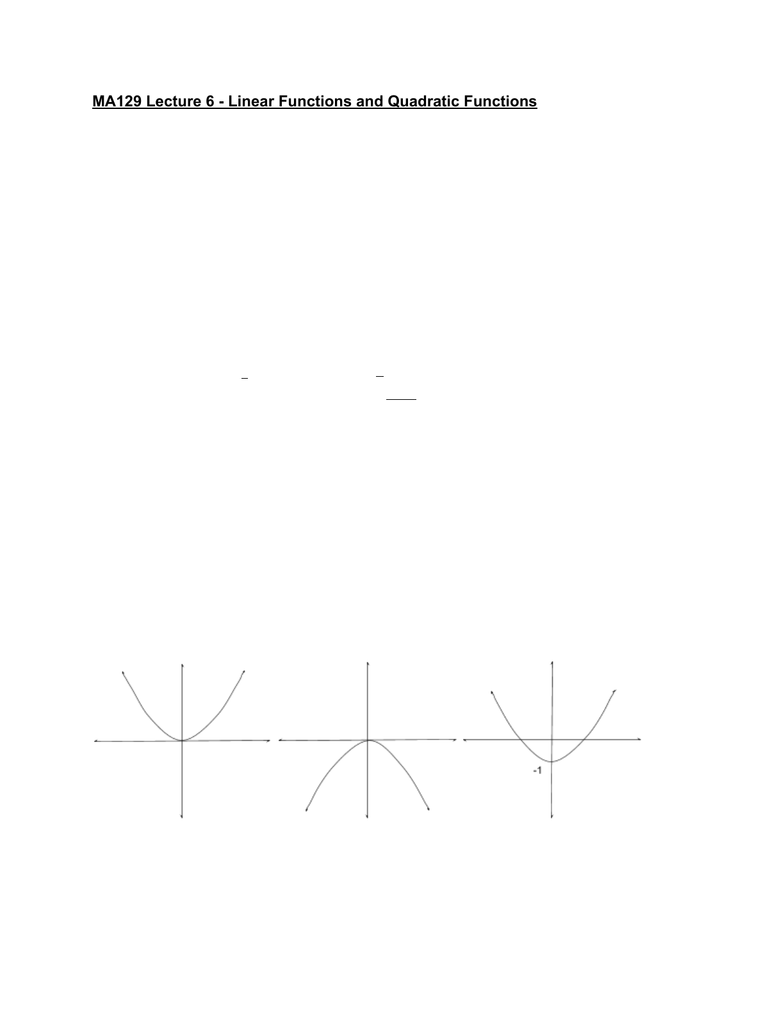# MA129 Lecture 6: Linear Functions

78 views4 pages
School
Department
CourseJanuary 18, 2019
MA129 Lecture 6 - Linear Functions and Quadratic Functions
Recall: a function like is a rule that assigns output to an input. (Dr. Allison)(x)f
We can also write:
or (x)y=f(x)y:xf
Note: Function evaluation is very important for calculus.
Eg. (x)xf = 3 − 2 (Dr. Allison)
(− ) (− )f2 = 3 2 2
(− ) f2 = 6 2 = 8
While (x) (x)f+h= 3 + h 2
In this case we make in x x+h(x)f
Note: the symbol → means “become”
Eg. If and (x)g=x2+ 3x(h)g=h2+ 3h
Following this, (x)g+h= (x)+ h2+ 3x+h
Note: in general, (x) = (x) (h)g+h/g+g
Quadratic Functions
where:(x)x xf =a2+b+c
=a/ 0
, , ε Ra b c
Degree of is 2(x)f
is a polynomialf (Dr. Allison)
Shape of a quadratic function is a parabola
y=x2y=x2y=x2 1
Unlock document

This preview shows page 1 of the document.
Unlock all 4 pages and 3 million more documents.

Already have an account? Log in

# Get access

Grade+
\$10 USD/m
Billed \$120 USD annually
Homework Help
Class Notes
Textbook Notes
40 Verified Answers
Study Guides
1 Booster Class
Class+
\$8 USD/m
Billed \$96 USD annually
Homework Help
Class Notes
Textbook Notes
30 Verified Answers
Study Guides
1 Booster Class